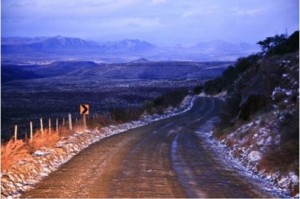A motorist plans to drive to Cradock. Event X is that he arrives at his destination in less than 3 hours. He estimates that the probability of it snowing is 1/6 and the probability of event X if it snowed is 1/10.

What is the probability that it snows and he gets there in less than 3 hours?

A motorist plans his journey hoping to arrive at his destination in less than 3 hours (event X). He estimates that the probabilities of the events: dry weather D, rain R and snow S to be 1/3, 1/2 and 1/6 respectively. The probabilities of event X in these conditions is 3/4, 2/5 and 1/10 respectively. What is the probability of event X?